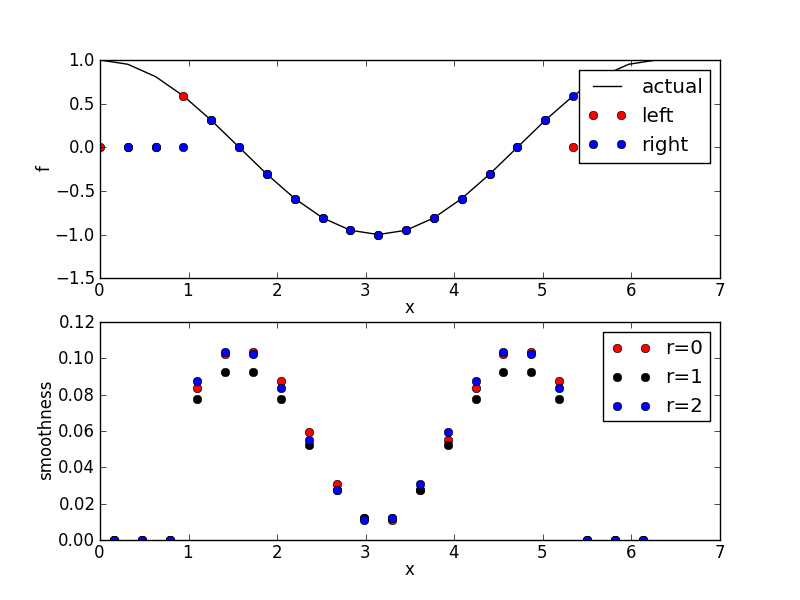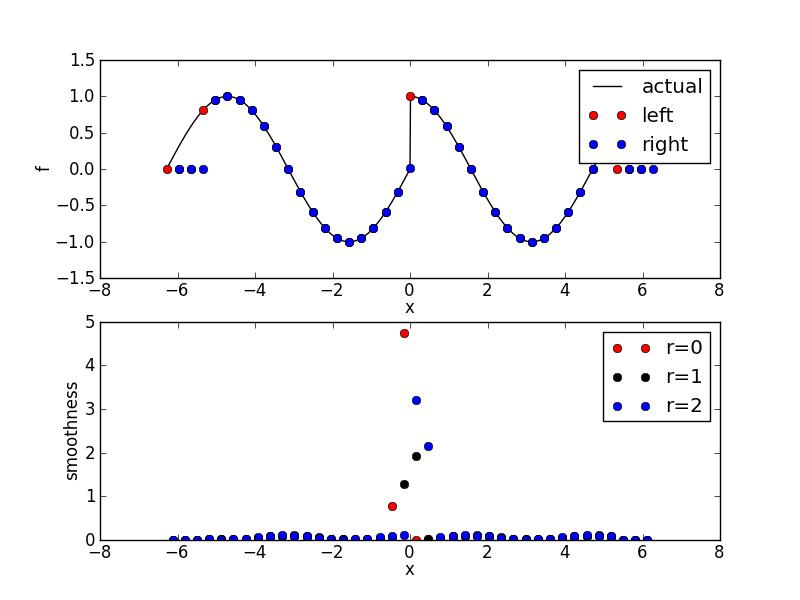# WENO toolkit¶

## WENO reconstructions¶

High-order WENO reconstructions for 1d arrays of cell-average quantities can be computed with the pyweno.weno module.

For example, to reconstruct sin(x) at the left edge of each cell to fifth order accuracy:

>>> import numpy as np
>>> import pyweno.weno
>>> x = np.linspace(0.0, 2*np.pi, 21)
>>> f = (np.cos(x[1:]) - np.cos(x[:-1])) / (x - x)
>>> q = pyweno.weno.reconstruct(f, 5, 'left')


### Smooth reconstruction¶

Here we reconstruct sin(x) at the left and right edges of each cell to fifth order accuracy and plot the results:The code to generate the above is in smooth.py.

### Discontinuous reconstruction¶

Here we reconstruct a discontinuous function (sin(x) for x<0, cos(x) for x>0) at the left and right edges of each cell to fifth order accuracy and plot the results:The code to generate the above is in discontinuous.py.

## Version information¶

Here we obtain the version of PyWENO:

>>> import pyweno.version
>>> pyweno.version.version()
>>> pyweno.version.git_version()